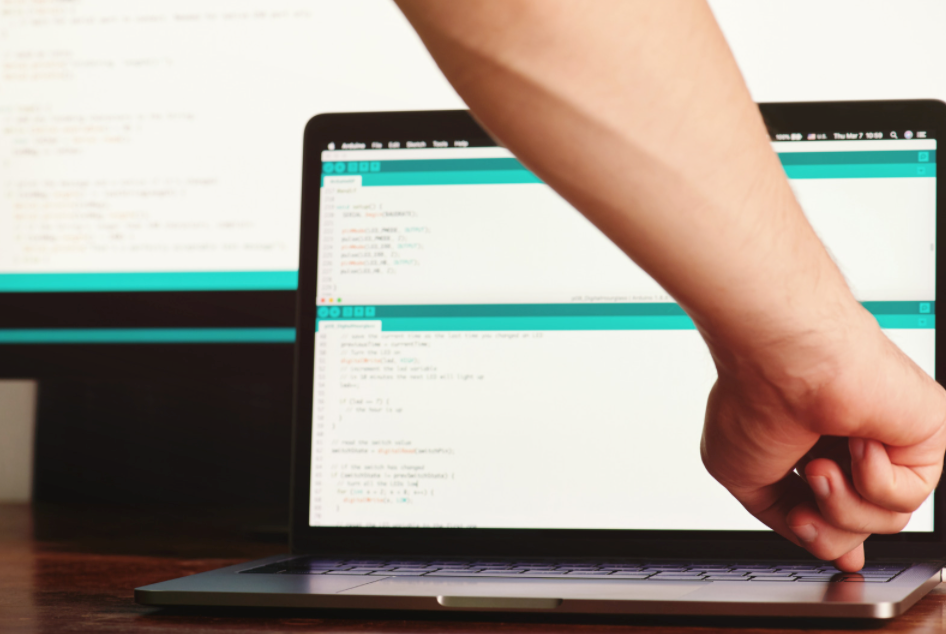Search

# Learning to debug code: A Guide for K-12 Students (PART II)

Updated: Apr 11In our first code debugging guide post, we described different types of bugs and best practices for debugging deterministic crashes by focusing on the reported error message. In this blog, we describe another technique that should be in your toolbox - understanding what your code is actually doing (which may be different from what you thought it was doing when you wrote it!)

This type of debugging can be used for every type of bug, but it is particularly useful for bugs where the code is failing in a deterministic way (i.e. doing something you did not expect and doing it every time). Here is a simple example. You wanted to add up all the numbers between 1 and 5. The code below, however, returns 10 when the sum of numbers (1,2,3,4,5) is not 10 but 15. What is wrong?

```count = input("How many numbers to add up? ")
sum = 0
for i in range(0,int(count)):
sum = sum + i
print(sum)

```

When this code is run, the output is

```How many numbers to add up? 5
10

```

Now - this code is simple enough that you may already know what is wrong - but using a step-by-step process to debug it is still a good idea since that same process will help debug errors that are less obvious. What are the steps?

• First, we know that the sum is calculated by the loop. Let's confirm for ourselves that the loop does what we expect. To do this, we will add a print statement inside the loop that prints out every number that is being added at each iteration (run) of the loop. Once we add this, the code looks like this:

```count = input("How many numbers to add up? ")
sum = 0
for i in range(0,int(count)):
print(i)
sum = sum + i
print(sum)

```

• Now, let's run this program. We can see that the prints show

```How many numbers to add up? 5
0
1
2
3
4
10

```

• So - this is not what we expect! We wanted the numbers 1-5 but we are getting 0-4. Why is this?

• Now - let’s look at the loop more carefully. We can see that the way it is written, it will only run till 4. Now - how to fix this? We can set the range of the loop